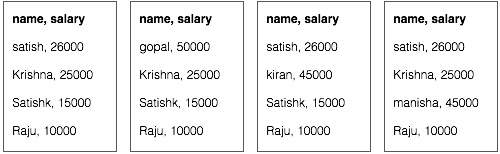# MapReduce - Algorithm

The MapReduce algorithm contains two important tasks, namely Map and Reduce.

• The map task is done by means of Mapper Class
• The reduce task is done by means of Reducer Class.

Mapper class takes the input, tokenizes it, maps and sorts it. The output of Mapper class is used as input by Reducer class, which in turn searches matching pairs and reduces them.MapReduce implements various mathematical algorithms to divide a task into small parts and assign them to multiple systems. In technical terms, MapReduce algorithm helps in sending the Map & Reduce tasks to appropriate servers in a cluster.

These mathematical algorithms may include the following −

• Sorting
• Searching
• Indexing
• TF-IDF

## Sorting

Sorting is one of the basic MapReduce algorithms to process and analyze data. MapReduce implements sorting algorithm to automatically sort the output key-value pairs from the mapper by their keys.

• Sorting methods are implemented in the mapper class itself.

• In the Shuffle and Sort phase, after tokenizing the values in the mapper class, the Context class (user-defined class) collects the matching valued keys as a collection.

• To collect similar key-value pairs (intermediate keys), the Mapper class takes the help of RawComparator class to sort the key-value pairs.

• The set of intermediate key-value pairs for a given Reducer is automatically sorted by Hadoop to form key-values (K2, {V2, V2, …}) before they are presented to the Reducer.

## Searching

Searching plays an important role in MapReduce algorithm. It helps in the combiner phase (optional) and in the Reducer phase. Let us try to understand how Searching works with the help of an example.

### Example

The following example shows how MapReduce employs Searching algorithm to find out the details of the employee who draws the highest salary in a given employee dataset.

• Let us assume we have employee data in four different files − A, B, C, and D. Let us also assume there are duplicate employee records in all four files because of importing the employee data from all database tables repeatedly. See the following illustration.• The Map phase processes each input file and provides the employee data in key-value pairs (<k, v> : <emp name, salary>). See the following illustration.• The combiner phase (searching technique) will accept the input from the Map phase as a key-value pair with employee name and salary. Using searching technique, the combiner will check all the employee salary to find the highest salaried employee in each file. See the following snippet.

```<k: employee name, v: salary>
Max= the salary of an first employee. Treated as max salary

if(v(second employee).salary > Max){
Max = v(salary);
}

else{
Continue checking;
}
```

The expected result is as follows −





• Reducer phase − Form each file, you will find the highest salaried employee. To avoid redundancy, check all the <k, v> pairs and eliminate duplicate entries, if any. The same algorithm is used in between the four <k, v> pairs, which are coming from four input files. The final output should be as follows −

```<gopal, 50000>
```

## Indexing

Normally indexing is used to point to a particular data and its address. It performs batch indexing on the input files for a particular Mapper.

The indexing technique that is normally used in MapReduce is known as inverted index. Search engines like Google and Bing use inverted indexing technique. Let us try to understand how Indexing works with the help of a simple example.

### Example

The following text is the input for inverted indexing. Here T, T, and t are the file names and their content are in double quotes.

```T = "it is what it is"
T = "what is it"
T = "it is a banana"
```

After applying the Indexing algorithm, we get the following output −

```"a": {2}
"banana": {2}
"is": {0, 1, 2}
"it": {0, 1, 2}
"what": {0, 1}
```

Here "a": {2} implies the term "a" appears in the T file. Similarly, "is": {0, 1, 2} implies the term "is" appears in the files T, T, and T.

## TF-IDF

TF-IDF is a text processing algorithm which is short for Term Frequency − Inverse Document Frequency. It is one of the common web analysis algorithms. Here, the term 'frequency' refers to the number of times a term appears in a document.

### Term Frequency (TF)

It measures how frequently a particular term occurs in a document. It is calculated by the number of times a word appears in a document divided by the total number of words in that document.

```TF(the) = (Number of times term the ‘the’ appears in a document) / (Total number of terms in the document)
```

### Inverse Document Frequency (IDF)

It measures the importance of a term. It is calculated by the number of documents in the text database divided by the number of documents where a specific term appears.

While computing TF, all the terms are considered equally important. That means, TF counts the term frequency for normal words like “is”, “a”, “what”, etc. Thus we need to know the frequent terms while scaling up the rare ones, by computing the following −

```IDF(the) = log_e(Total number of documents / Number of documents with term ‘the’ in it).
```

The algorithm is explained below with the help of a small example.

### Example

Consider a document containing 1000 words, wherein the word hive appears 50 times. The TF for hive is then (50 / 1000) = 0.05.

Now, assume we have 10 million documents and the word hive appears in 1000 of these. Then, the IDF is calculated as log(10,000,000 / 1,000) = 4.

The TF-IDF weight is the product of these quantities − 0.05 × 4 = 0.20.